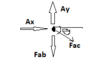# Signs of Tensile and Compressive Stresses

• a1234
in that situation, if the force is really compressive, you end up with a negative value for the stress.

#### a1234

TL;DR Summary
Signs (directions) of forces on FBDs are not matching up with the conventions used for tensile and compressive stresses
I have recently learned that, by convention, axial tensile stresses are positive and axial compressive stresses are negative. I am having some trouble incorporating this information into my FBDs that are used to find the axial forces leading to these stresses.

For instance, from the attached FBD, I ended up finding Fab to be -500 N, which indicates that the force is actually compressive (directed toward point A) and not tensile as drawn in the FBD. However, if I had originally drawn Fab to be in compression, then wouldn't I find Fab = +500 N?

If I had drawn the latter kind of FBD and used Fab = +500 N to find the axial stress in Fab, I would end up with a positive value for the stress, which I know is wrong because the stress is compressive. In this case, how can I make the stress a negative value?

Thanks!

#### Attachments

•FBD of Point.PNG
891 bytes · Views: 272
a1234 said:
Summary: Signs (directions) of forces on FBDs are not matching up with the conventions used for tensile and compressive stresses

I have recently learned that, by convention, axial tensile stresses are positive and axial compressive stresses are negative. I am having some trouble incorporating this information into my FBDs that are used to find the axial forces leading to these stresses.

For instance, from the attached FBD, I ended up finding Fab to be -500 N, which indicates that the force is actually compressive (directed toward point A) and not tensile as drawn in the FBD. However, if I had originally drawn Fab to be in compression, then wouldn't I find Fab = +500 N?

If I had drawn the latter kind of FBD and used Fab = +500 N to find the axial stress in Fab, I would end up with a positive value for the stress, which I know is wrong because the stress is compressive. In this case, how can I make the stress a negative value?

Thanks!
The plus and minus signs have multiple meanings in Physics and Engineering. Similar perhaps to your example, if you assume that a force acts down and you get a negative solution for that force, it means that you assumed the wrong direction for the force, and that it actually acts up. If you got a positive solution for that force, it means you assumed the correct direction for that force, even though it may be a compressive force.

Forget about actual signage for tension and compressive axial forces in a free body diagram...I mean how do you assign a positive or negative values to a force acting at a 45 degree angle?

The rule is this: if the force points inward toward the joint or member, it is a compressive force. If it points outward away from the joint or member, it is a tensile force. For the compression case , you call it a negative force, for the tension case, you call it a positive force, by convention. So don’t give it a sign until the end. If you assumed the direction of all forces correctly up front, you are going to get all positive numbers. Only after you determine if it is a pushing force or pulling force can you then give it a sign. Or don’t give it a sign, just say for sample that ‘the force is 10 N compressive’.

Thanks for the explanation. This makes a lot of sense.

Somehow, I assumed that the signs were supposed to "carry over" to the stresses in compression and tension, and I didn't think of them as having separate meanings.

You are welcome. Yeah, the minus sign is always a killer...

## 1. What is the definition of tensile stress?

Tensile stress is the force per unit area that is applied to a material in the direction of its length, causing it to elongate or stretch.

## 2. How can tensile stress be measured?

Tensile stress can be measured using a device called a tensile tester, which applies a pulling force to a material and measures the resulting elongation or deformation.

## 3. What are some common signs of tensile stress in materials?

Some common signs of tensile stress in materials include cracking, fracturing, and elongation or stretching beyond its original shape.

## 4. What is the difference between tensile stress and compressive stress?

Tensile stress is a pulling force that elongates a material, while compressive stress is a pushing force that shortens a material. They are both types of internal stresses that can occur in materials.

## 5. How can tensile and compressive stresses affect the strength of a material?

Tensile and compressive stresses can weaken a material by causing it to deform or break. However, a certain amount of stress is necessary for materials to function properly and can even increase their strength if they are designed to withstand it.